# ADO.NET is Fun (Part I)

### Dive into ADO.NET and see what it can offer in the realm of creating distributed data-sharing applications.

· Database Zone · Tutorial
Save
3.42K Views

Editor's note: Labeled references can be found at the bottom of the article.

### Introduction

ADO.NET is a set of classes  that expose data access services for .NET Microsoft Framework programmers. ADO.NET provides a rich set of  components for creating distributed data-sharing applications .

Visually, an ADO.NET instance will have two parts, and they are shown in the below figure (based was based on reference ):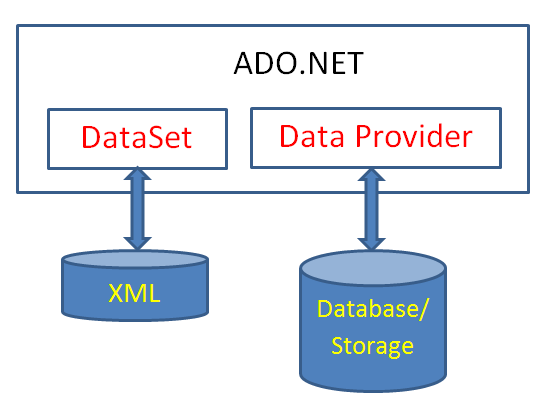DataSet contains two collections: DataTableCollection contains zero or more DataTable objects and DataRelationCollection contains zero or more DataRelation objects. Like a relational database, DataTable objects are like tables and DataRelation objects will set the relationship among those tables. We can see DataSet figure looks like this: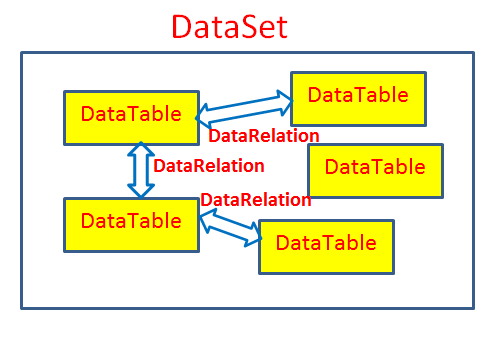A DataTable object is made up by DataColumn and DataRow object. Like a data table is made up of columns and data rows, the DataColumn object and DataRow object will define the column and data row for the DataTable object.

When a data table is created, it can be viewed by many other ways. This is easy understand because there are many users who will use your data table for their other purposes. The DataView object will be used for this purpose.

DataTable and DataView figure can be shown like this: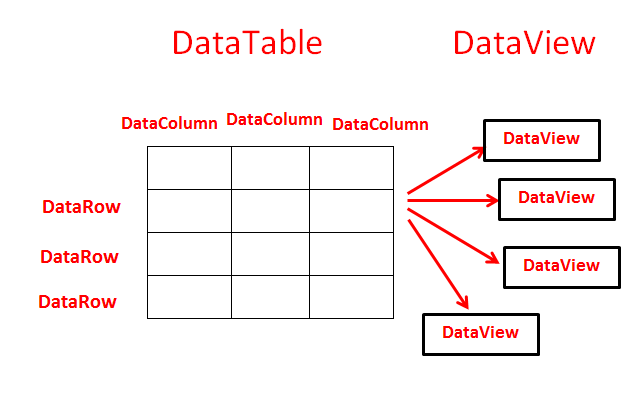In this article, I will explain about the DataTable class, the DataColumn class, the DataRow class, properties, methods by using VB code examples. You can read documents about these subjects in detail at , ,  (or other sources).

## Students Table Example

Assume that I want to create a data table for storing students' information and its name is Students. Each student has two properties: ID (student code) and Name (name of student).

I create a Windows Forms Application project, and it has a form that looks like this (includes controls and their properties):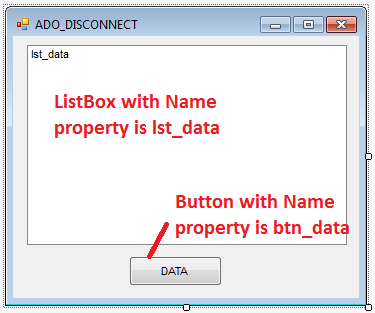And I also assume that all the following code examples are put into the Click event of the DATA button.

## How to create a data table

There are some steps to create a data table as follows:

Step 1: Create a data table object (in this case, the Students table) by using the DataTable class.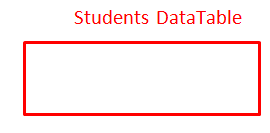VB code:

``Dim st As New DataTable("Students")``

And if we want to see name of this table (i.e Students), we can use the TableName property as follows:

``st.TableName``

I display the table name in the listbox:

``lst_data.Items.Add("Table Name: " & st.TableName)``

Above form looks like (after clicking the Data button):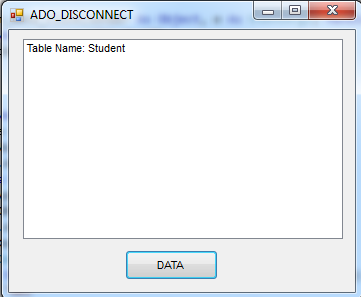Step 2
: After creating the data table, we will add columns (ID and Name) to it. In database work, we call this is the process of creating schema. The DataColumn class is used for creating columns, and when columns are added to the table, we must choose one or more columns to set the primary key (in this our case is ID) by using PrimaryKey property of  the DataTable class.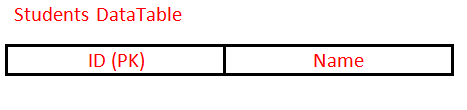VB code:

``````Dim id As New DataColumn("ID")
id.DataType = GetType(String)
id.AllowDBNull = False
Dim name As New DataColumn("Name")
name.DataType = GetType(String)
name.AllowDBNull = True

Choose the primary key column (ID):

``st.PrimaryKey = New DataColumn() {id}``

Display table's columns in the listbox

``````For Each dc As DataColumn In st.Columns
Next``````

Or:

``````Dim sColumns As New System.Text.StringBuilder()
For Each dc As DataColumn In st.Columns
sColumns.AppendFormat("{0,15}", dc.ColumnName)
Next

The form looks like this: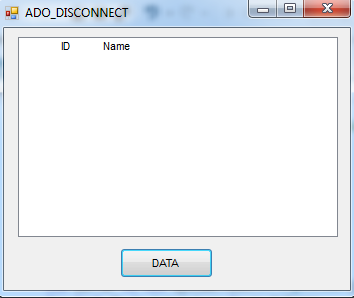Step 3
: When we have created the data table with columns and we also have chosen the primary key column, we will add actual data rows into the table by using the DataRow class. Here's our work so far: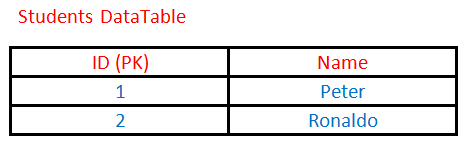VB code:

``````st.Rows.Add("1", "Peter")

Display the data in the listbox

``````For Each r In st.Rows
lst_data.Items.Add(r.Item(0) & "  " & r.Item(1))
Next r``````

Now our form looks like this: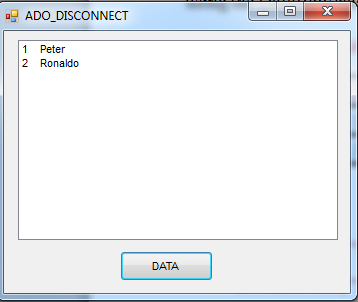Step 4: View a table's data by using the DataView class. Using a DataView, we can expose the data in a table with different sort orders (by using Sort property), or we can filter the data based on a filter expression (by using RowFilter property) .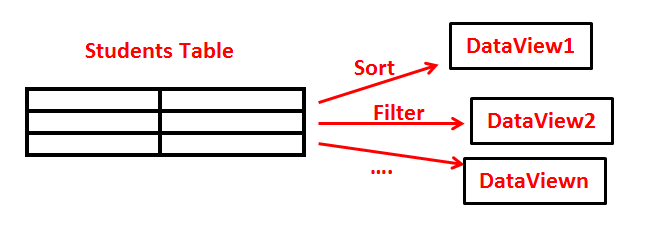Suppose I want to display the data in the Students table based on the ID column in descending order, code can be shown by using the Sort property:

``````Dim dv As New DataView(sv) ' creating new dataview object
Dim i
dv.Sort = "ID DESC" ' rows are sorted on the ID column in descending order
For i = 0 To dv.Count - 1
lst_data.Items.Add(dv(i)("ID") & "   " & dv(i)("Name"))
Next``````

Our form now looks like this:And now, I want the data in Students to be shown with a compound sort on the ID column in ascending order and on the Name column in descending order. The code looks like this:

``````Dim dv As New DataView(sv) ' creating new dataview object
Dim i
dv.Sort = "ID ASC, Name DESC" ' rows are sorted on the ID column
'in ascending order and on the Name in descending order
For i = 0 To dv.Count - 1
lst_data.Items.Add(dv(i)("ID") & "   " & dv(i)("Name"))
Next``````

And we can filter the data in Students. For example, we can display a filter on the Name column for students beginning with "R":

``````Dim dv As New DataView(sv) ' creating new dataview object
Dim i
dv.RowFilter = "Name like 'R%'" ' a filter on the Name column
'for students beginning with the R letter
For i = 0 To dv.Count - 1
lst_data.Items.Add(dv(i)("ID") & "   " & dv(i)("Name"))
Next``````

Our form looks like this: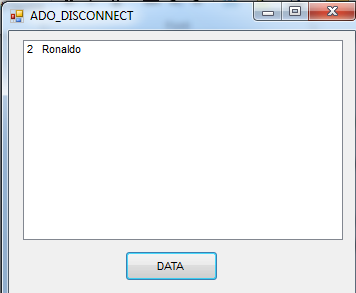## Some important methods

Beside the Add method, when we work on the DataTable object, we also meet other methods such as AcceptChanges, RejectChanges, Delete, Copy, Clone. Their functions are shown in detail in , , and . Some examples:

### Example of AcceptChanges:

``````st.Rows.Add("1", "Peter")
'update new rows
st.AcceptChanges()
For Each r In st.Rows
lst_data.Items.Add(r.Item(0) & "  " & r.Item(1))
Next r``````

### Example of RejectChanges:

``````st.Rows.Add("1", "Peter")
'reject new rows
st.RejectChanges()
For Each r In st.Rows
lst_data.Items.Add(r.Item(0) & "  " & r.Item(1))
Next r``````

### Example of the Delete method:

``````lst_data.Items.Add("******before deleting*************")
For Each r In st.Rows
lst_data.Items.Add(r.Item(0) & "  " & r.Item(1))
Next r
st.Rows(1).Delete()'delete second row
st.AcceptChanges()
For Each r In st.Rows
lst_data.Items.Add(r.Item(0) & "  " & r.Item(1))
Next r``````

### Example of the Copy method:

``````st.Rows.Add("1", "Peter")
'copy data and schema to new table
Dim copyofStudentTable As DataTable = st.Copy()
For Each r_copy As DataRow In copyofStudentTable.Rows
lst_data.Items.Add(r_copy.Item(0) & "  " & r_copy.Item(1))
Next r_copy``````

### Example of the Clone and ImportRow methods:

``````st.Rows.Add("1", "Peter")
'copy just schema
Dim cloneofStudentTable As DataTable = st.Clone()
'import the third row of the Student Table into the clone table
cloneofStudentTable.ImportRow(st.Rows(2))
For Each r_clone As DataRow In cloneofStudentTable.Rows
Next r_clone``````

### The RowState property

We can use this property to retrieve the current state of a data row. Some RowState values can be shown in detail in , , and , such as Detached, Added, Unchanged, Modified, and Deleted.

The following example shows the Rowstate transitions at different times in the DataRow object's life:

``````Dim dr As DataRow = sv.NewRow() ' Rowstate: Detached
Dim rs1 As String = String.Format("Rowstate 1:{0}", dr.RowState)
dr("ID") = "1" 'Rowstate: Detached
Dim rs2 As String = String.Format("Rowstate 2:{0}", dr.RowState)
dr("Name") = "Peter"
Dim rs3 As String = String.Format("Rowstate 3:{0}", dr.RowState)
sv.AcceptChanges()'Rowstate: Unchanged
Dim rs4 As String = String.Format("Rowstate 4:{0}", dr.RowState)
dr("ID") = "3"'Rowstate: Modified
Dim rs5 As String = String.Format("Rowstate 5:{0}", dr.RowState)
sv.RejectChanges()'Rowstate: Unchanged
Dim rs6 As String = String.Format("Rowstate 6:{0}", dr.RowState)
dr.Delete()'Rowstate: Deleted
Dim rs7 As String = String.Format("Rowstate 7:{0}", dr.RowState)
Dim rs = rs1 + vbCrLf + rs2 + vbCrLf + rs3 + vbCrLf + rs4 + vbCrLf + rs5 + vbCrLf + rs6 + vbCrLf + rs7 + vbCrLf
MsgBox(rs)``````

Result: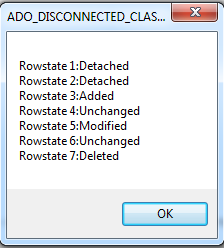### Conclusion

In this article (Part I), I have introduced some ADO.NET classes such as DataTable, DataColumn, DataRow, and DataView. In my next articles, I will introduce some other classes such as DataSet and DataRelation, and I will also introduce some techniques such as serialization, data binding, etc. I hope you find my article helpful!

### Refferences

 www.msdn.microsoft.com

 Glenn Johnson, Accessing Data with Microsoft.NET Framework 4

 Tim Patrick, Microsoft ADO.NET 4 Step by Step

Topics: